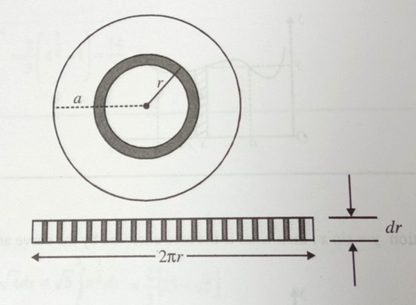# NEET Physics Mathematical Tools Questions SolvedCalculate the area of disk of radius 'a' using integration

1. $\frac{{\mathrm{\pi a}}^{2}}{2}$

2. ${\mathrm{\pi a}}^{2}$

3. $\frac{3}{2{\mathrm{\pi a}}^{2}}$

4. $2{\mathrm{\pi a}}^{2}$

Concept Videos :-

#16 | Calculus: Differential Equation
#19 | Numericals

Concept Questions :-

Integration

A disk of radius a can be assumed to be made of infinite concentric rings each of small thickness dr. Now consider a ring of inner radius r and outer radius r+dr. If we cut out this smalll ring, cut it along the radius and straighten it out, we obtain a rectangle of length $2\mathrm{\pi r}$ and thickness dr.Total area of disk  [Since radius of innermost ring is zero and that of outer most is a]

=

Difficulty Level:

• 16%
• 66%
• 4%
• 16%
Crack NEET with Online Course - Free Trial (Offer Valid Till September 21, 2019)